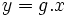Homomorphism from automorphism group of connected groupoid to outer automorphism group at a point

Consider a connected groupoid with$X$ its collection of points and$P(x,y)$ denoting the paths between points$x,y \in X$. Let$x \in X$ be a point. Denote by$A$ the group of automorphisms of the groupoid$X$, and by$G_x$ the group$P(x,x)$ under composition. Then, there is a canonical homomorphism:$A \to \operatorname{Out}(G_x)$
• Given$g \in A$, pick a path from$x$ to$y = g.x$. Call this path$p$.
• Consider now the map sending$h \in G_x$ to$p^{-1} \circ (g.h) \circ p$. Note that this map is an automorphism of the group$G_x$.
• Now, the automorphism we got depended on the choice of path from$x$ to$y$. Choosing a different path gives an automorphism that differs from the original one by an inner automorphism. Hence, we get a well-defined element of$\operatorname{Out}(G_x)$.